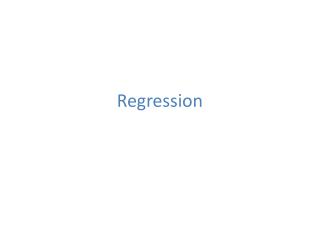Download PresentationRegression

# Regression - PowerPoint PPT PresentationDownload Presentation## Regression

- - - - - - - - - - - - - - - - - - - - - - - - - - - E N D - - - - - - - - - - - - - - - - - - - - - - - - - - -
##### Presentation Transcript

1. Regression

2. Population Covariance and Correlation

3. Sample Correlation

4. Sample Correlation -.04 .98 -.79

5. Linear Model DATA REGRESSION LINE

6. (Still) Linear Model DATA REGRESSION CURVE

7. Parameter Estimation Minimize SSE over possible parameter values

8. Fitting a linear model in R

9. Fitting a linear model in R Intercept parameter is significant at .0623 level

10. Fitting a linear model in R Slope parameter is significant at .001 level, so reject

11. Fitting a linear model in R Residual Standard Error:

12. Fitting a linear model in R R-squared is the correlation squared, also % of variation explained by the linear regression

13. Create a Best Fit Scatter Plot

14. Add X and Y Labels

15. Inspect Residuals

16. Multiple Regression Example: we could try to predict change in diameter using both change in height as well as starting height and Fertilizer

17. Multiple Regression • All variables are significant at .05 level • The Error went down and R-squared went up (this is good) • Can even handle categorical variables

18. Regression w/ Machine Learning point of view

19. Regression w/ Machine Learning point of view Music Year Timbre (90 attributes) http://archive.ics.uci.edu/ml/datasets/YearPredictionMSD • Let’s “train” (fit) different models to a training data set • Then see how well they do at predicting a different “validation” data set (this is how ML competitions on Kaggle work)

20. Regression w/ Machine Learning point of view • Create a random sample of size 10000 from original 515,345 songs • Assign first 5000 to training data set, second 5000 are saved for validation

21. Regression w/ Machine Learning point of view • Fit linear model and generalized boosting regression model (other popular choices include random forests and neural networks) • The period after the tilde denotes we will use all 91 variables for training, the –V1 throws out V1 (since this is what we’re predicting)

22. Regression w/ Machine Learning point of view • Next we make predictions for the validation data set • We compare the models by calculating the sum of squares error (SSE) for each model

23. Regression w/ Machine Learning point of view